Magnequench Technical Resource Website, a subsite of www.neomaterials.com

## Factors Affecting Size of Motor – Part 2

Motor output power:

Pout=KBav acD2 LNs

where,

Pout = Output power (W)
K = Constant
Bav = Average airgap flux density (T)
D = Rotor diameter (m), L= Length of rotor (m)
Ns = Motor speed (rpm)

For the same output power, the size of the motor can be reduced by:

1. Increase in magnetics/magnetic loading (i.e. Increase in magnet Br and hence the Bav)
2. Increase in motor speed (Ns)
3. Increase in winding current or no. of turns/coil (i.e. Increase in ac)

## Increase In Motor Speed

For a given output power, if the motor is designed to operate at double the operational speed, only half the torque is required.

Pout1=T1 ω1, Pout2=T2 ω2,
If Pout1=Pout2, ω2=2ω1, T2=0.5T1
where, T=Torque and ω =2πNs60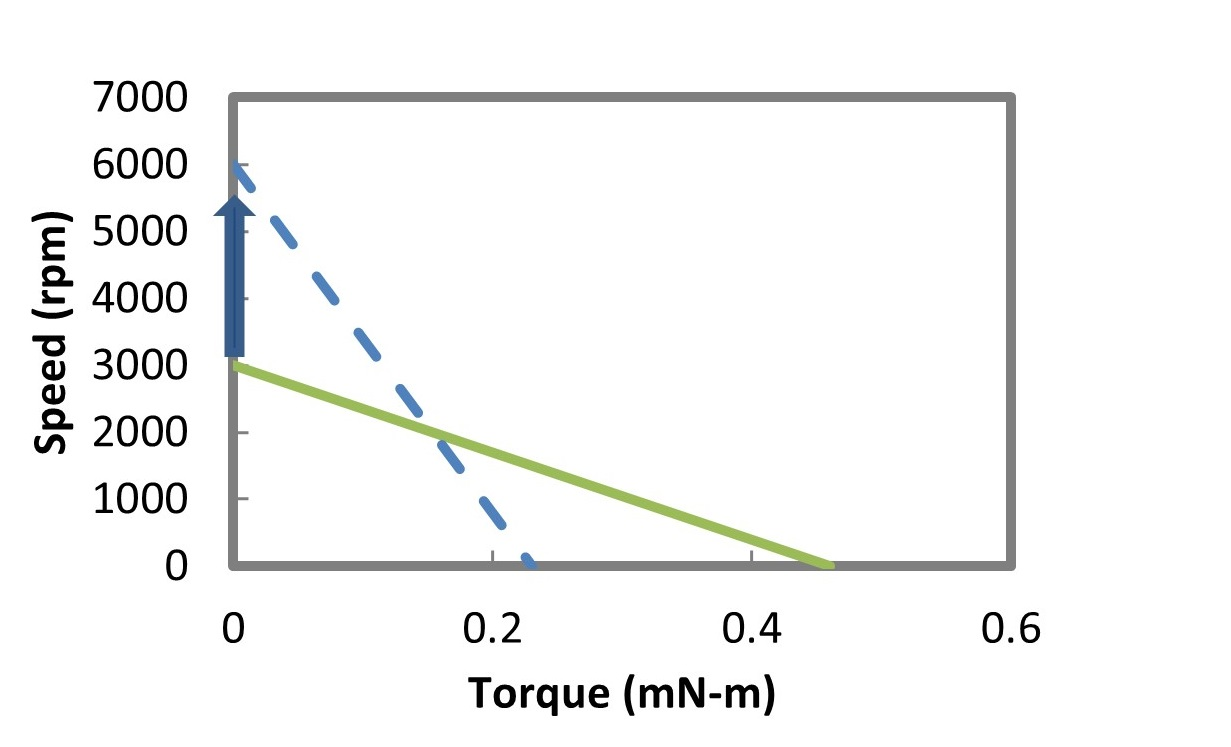This equation dictates that a higher speed motor is always smaller (and lighter) for any given Pout.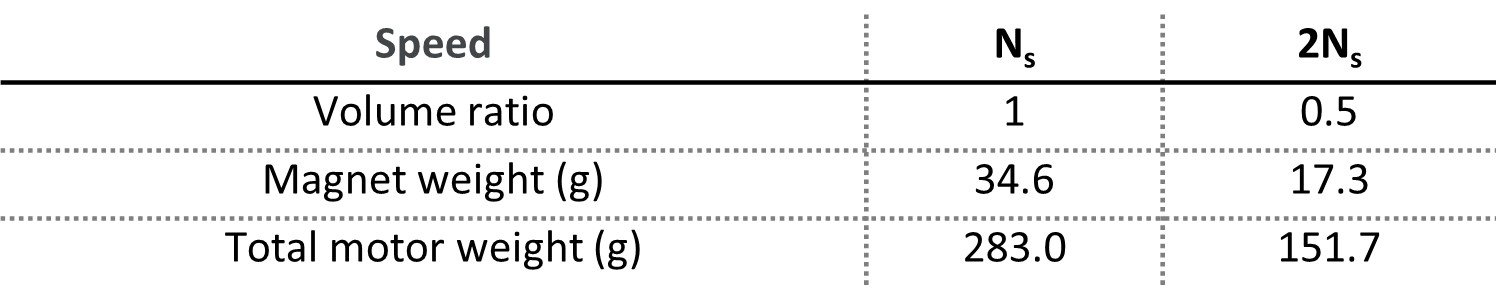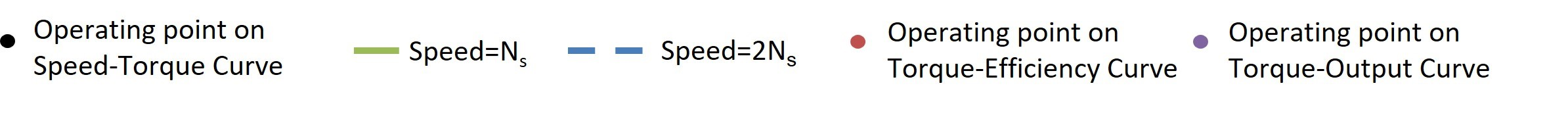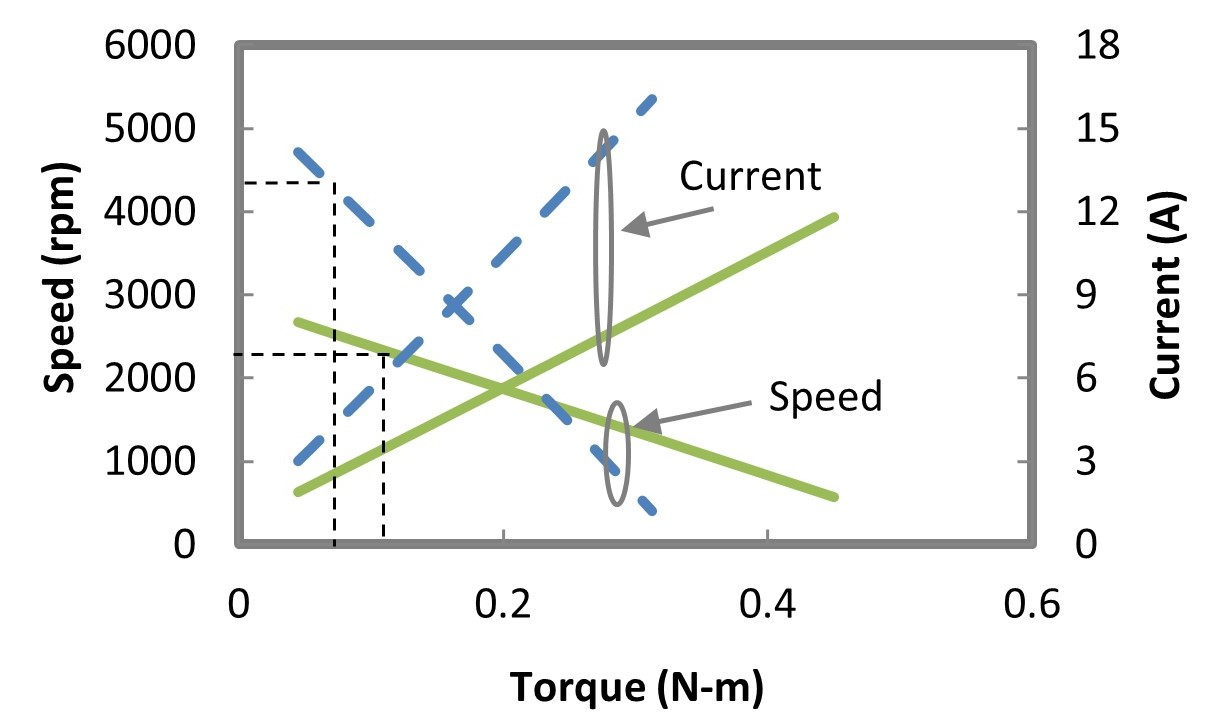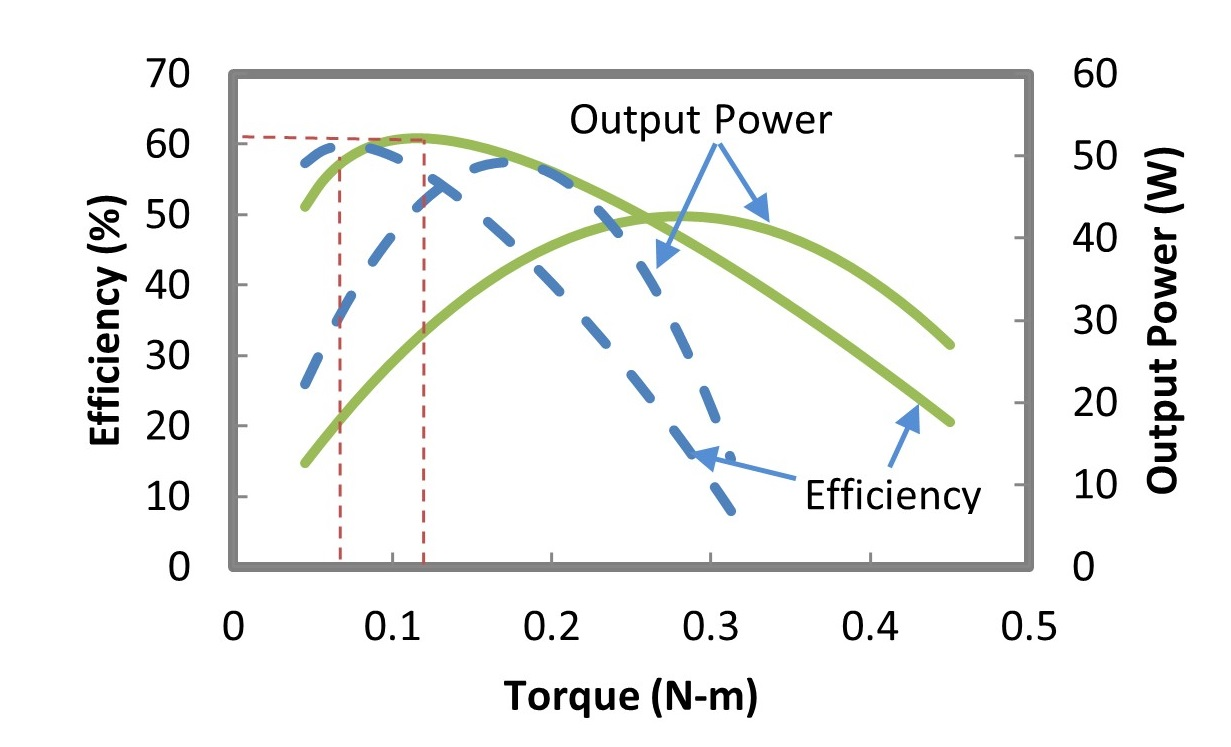Following considerations should be made while deciding the motor speed:

• Influence on gear system: e.g. Gear system with higher gear ratio may become mechanically weak
• Influence on noise and vibration: e.g. Higher motor speed may lead to higher noise and vibration
• Influence on core losses: e.g. Higher motor speed may lead to higher core losses
• Influence on brushes: e.g. Higher speed may lead to sparking at brush and commutator surface